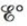# Calculate values for the following g cells. Which reactions are spontaneous as written (under standard conditions)? Balance the equations. Standard reduction potentials are found in Table 17-1. a. MnO 4 − ( a q ) + I − ( a q ) → I 2 ( a q ) + Mn 2+ ( a q ) b. MnO 4 − ( a q ) + F − ( a q ) → F 2 ( a q ) + Mn 2+ ( a q )### Chemistry: An Atoms First Approach

2nd Edition
Steven S. Zumdahl + 1 other
Publisher: Cengage Learning
ISBN: 9781305079243

#### Solutions

Chapter
Section### Chemistry: An Atoms First Approach

2nd Edition
Steven S. Zumdahl + 1 other
Publisher: Cengage Learning
ISBN: 9781305079243
Chapter 17, Problem 47E
Textbook Problem
10 views

## Calculatevalues for the following g cells. Which reactions are spontaneous as written (under standard conditions)? Balance the equations. Standard reduction potentials are found in Table 17-1.a. MnO 4 − ( a q ) + I − ( a q ) → I 2 ( a q ) + Mn 2+ ( a q ) b. MnO 4 − ( a q ) + F − ( a q ) → F 2 ( a q ) + Mn 2+ ( a q )

(a)

Interpretation Introduction

Interpretation:

The reaction taking place in two different galvanic cells is given. The E° of the given galvanic cells, the spontaneity of the reactions and balanced chemical equation for each cell is to be stated.

Concept introduction:

The galvanic cell converts chemical energy into electrical energy while the electrolytic cell converts electrical energy into chemical energy.

The species at anode undergoes oxidation while the species at cathode undergoes reduction and the electrons generated at the anode are transferred through wire to the cathode.

To determine: The set up of the cell given in the chemical equation and the direction of electron flow, identification of the cathode and anode and balanced chemical equation.

### Explanation of Solution

The value of E°cell is calculated as 0.97V_ .

The reaction taking place at cathode is,

MnO4(aq)+8H+(aq)+5eMn2+(aq)+4H2O(l)E°red=1.51V

The reaction taking place at anode is,

2I(aq)I2(aq)+2eE°ox=0.54V

Multiply reduction half with a coefficient of 2 and oxidation half with a coefficient of 5 and add both the oxidation and reduction half-reaction,

2MnO4(aq)+16H+(aq)+10e2Mn2+(aq)+8H2O(l)10I(aq)5I2(aq)+10e

The final equation is,

2MnO4(aq)+16H+(aq)+10I(aq)2Mn2+(aq)+8H2O(l)+5I2(aq)

The overall cell potential is calculated as,

E°cell=E°ox+E°red=0.54V+1.51V=0.97V_

The value of E°cell is 0.97V_ .

The cell reaction is spontaneous in nature.

The relationship between cell potential and Gibbs free energy change is given by the formula,

ΔG°=nFE°cell

Where,

• ΔG° is the Gibbs free energy change at the standard conditions.
• n is the number of electrons involved in the reaction.
• F is the Faraday’s constant.
• E°cell is the cell potential at the standard condition.

When the value of E°cell comes out to be positive then ΔG° becomes negative. Therefore it leads to a spontaneous reaction.

The balanced chemical equation for given reaction (a) is,

2MnO4(aq)+16H+(aq)+10I(aq)2Mn2+(aq)+8H2O(l)+5I2(aq)

The reduction half cell reaction is,

MnO4(aq)Mn2+(aq)

The change in the oxidation number of manganese is from +7 to +2

(b)

Interpretation Introduction

Interpretation:

The reaction taking place in two different galvanic cells is given. The E° of the given galvanic cells, the spontaneity of the reactions and balanced chemical equation for each cell is to be stated.

Concept introduction:

The galvanic cell converts chemical energy into electrical energy while the electrolytic cell converts electrical energy into chemical energy.

The species at anode undergoes oxidation while the species at cathode undergoes reduction and the electrons generated at the anode are transferred through wire to the cathode.

To determine: The set up of the cell given in the chemical equation and the direction of electron flow, identification of the cathode and anode and the balanced chemical equation.

### Still sussing out bartleby?

Check out a sample textbook solution.

See a sample solution

#### The Solution to Your Study Problems

Bartleby provides explanations to thousands of textbook problems written by our experts, many with advanced degrees!

Get Started

Find more solutions based on key concepts
Give the molecular formula for each of the following structural formulas.

General Chemistry - Standalone book (MindTap Course List)

The polysaccharide that helps form the supporting structures of plants is cellulose maltose glycogen sucrose

Nutrition: Concepts and Controversies - Standalone book (MindTap Course List)

A student measures the length of a brass rod with a steel tape at 20.0C. The reading is 95.00 cm. What will the...

Physics for Scientists and Engineers, Technology Update (No access codes included)